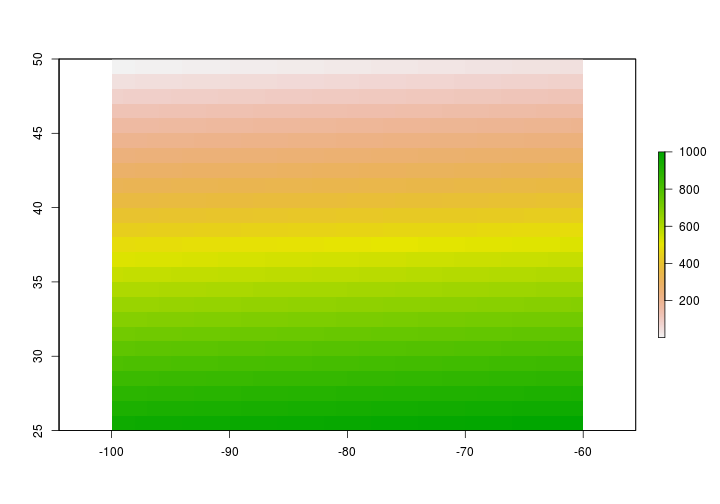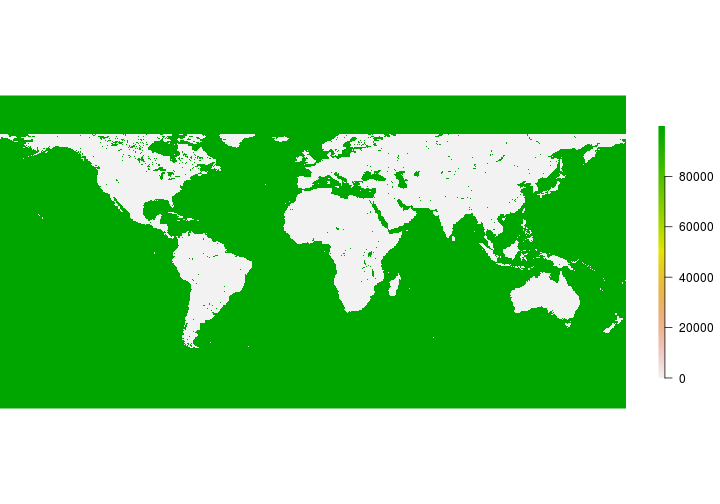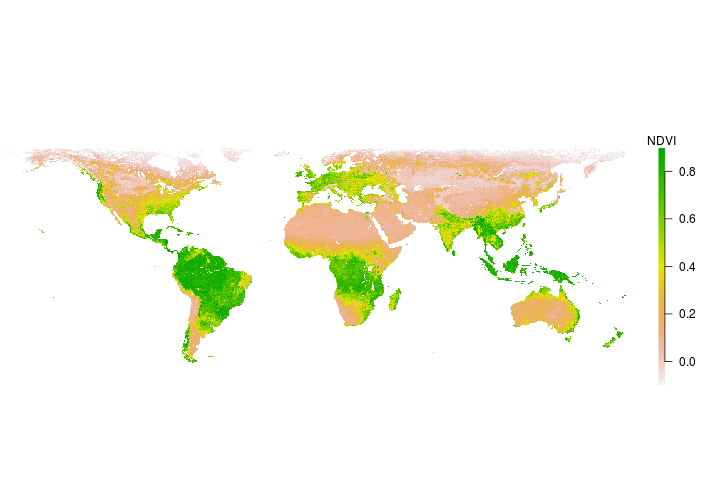# Introduction to raster data in R

This activity will introduce you to working with raster data in R.

R Skill Level: Intermediate - this activity assumes you have a working knowledge of `R`

Need to brush up on syntax and data classes in `R`? See R basics for a refresher.

# Objectives & Goals

Upon completion of this activity, you will:
• know how to create and write rasters
• know how to do basic calculations

### Required packages

To complete the following activity you will need the following packages installed: raster sp rgeos

#### Installing the packages

If installing these packages for the first time consider adding `dependencies=TRUE`
• `install.packages("raster",dependencies = TRUE)`
• `install.packages("rgdal",dependencies = TRUE)`
• `install.packages("rgeos",dependencies = TRUE)`
• `install.packages("sp",dependencies = TRUE)`

• Last modified: 2019-09-20 18:26:28

# What is a raster?

A raster is a spatially explicit matrix or `grid` where each cell represents a geographic location. Each cell represents a pixel on a surface. The size of each pixel defines the resolution or `res` of raster. The smaller the pixel size the finer the spatial resolution. The `extent` or spatial coverage of a raster is defined by the minima and maxima for both x and y coordinates.

Rasters can be created from the following data classes:

• Numeric
• Integer
• Categorical
• ## Raster formats

Raster data are stored in a variety of formats. The table below shows several commonly encountered file types. Use `raster::writeFormats()` to see the full list.

``````##      Name                  Long Name File Extension
## 1  raster                   R-raster           .grd
## 5     BIL               Band by Line           .bil
## 8   ascii                  Arc ASCII           .asc
## 22  GTiff                    GeoTIFF          .tiff
## 37 netCDF Network Common Data Format            .nc
``````

# Creating & writing rasters

## Raster data in R

Let’s begin by creating a raster from scratch. We’ll use the `raster` package to make an empty raster, set the `extent` and resolution (`res`) and assign values. Once we create a raster in R - we’ll take a closer look at the metadata and structure of rasters in `R`.

load the `raster` package if you haven’t already done so. If you need to install the `raster` package - see how to do that here

``````# load library
library(raster)
``````

Now that the `raster` library is loaded we can use the `raster()` function to create a raster in `R`.

``````# Create a raster from scratch using raster
firstRaster <- raster(xmn = -100,   # set minimum x coordinate
xmx = -60,    # set maximum x coordinate
ymn = 25,     # set minimum y coordinate
ymx = 50,     # set maximum y coordinate
res = c(1,1)) # resolution in c(x,y) direction
``````

Here is what that raster looks like in `R`

``````# Take a look at what the raster looks like
firstRaster
``````
``````## class      : RasterLayer
## dimensions : 25, 40, 1000  (nrow, ncol, ncell)
## resolution : 1, 1  (x, y)
## extent     : -100, -60, 25, 50  (xmin, xmax, ymin, ymax)
## crs        : +proj=longlat +datum=WGS84 +ellps=WGS84 +towgs84=0,0,0
``````

Notice that the object is of `class: RasterLayer` has 25 rows, 40 columns and 1000 cells. The resolution (`res`) is 1x1 degree. The raster’s `extent` ranges from -100 to -60 degrees longitude and 25 to 50 degrees latitude. The coordinate reference is WGS84 by default because `raster` recognized our inputs as degrees longitude/latitude. It doesn’t always do so.

Back to top

### Setting raster values

Currently there are no values associated with the raster layer we just created. It’s empty. We can assign values to the raster in a few ways. We’ll set the values of the raster using the `[]` convention. See the `setValues` function in the `raster` package for another way to set values of a raster. We’ll sequence values from 1 to the number of cells within the raster. You can extract the number of cells within a raster using the `ncell` function.
note - the number of values you supply needs to be equivilant to the number of cells in the raster. You can however provide `NA` values.

``````# Assign values to raster
firstRaster[] <- seq(from = 1, to = ncell(firstRaster),by = 1)

# Take a look at the raster now
firstRaster
``````
``````## class      : RasterLayer
## dimensions : 25, 40, 1000  (nrow, ncol, ncell)
## resolution : 1, 1  (x, y)
## extent     : -100, -60, 25, 50  (xmin, xmax, ymin, ymax)
## crs        : +proj=longlat +datum=WGS84 +ellps=WGS84 +towgs84=0,0,0
## source     : memory
## names      : layer
## values     : 1, 1000  (min, max)
``````

You should now notice there are a few new attributes to our raster object. We gained `data source:`, `names` and `values` fields. The `data source` attribute tells us that the raster information is stored in our memory. The `names` field gave a name to the `values` we provided. The `values` we supplied are now contained in the `values` field.

Now that the raster has values we can do a few things - like plot the raster.

``````plot(firstRaster)
``````Back to top

### Reading rasters from file

The `raster()` function within the `raster` package can also be used to read in a raster from file. Let’s read in some Normalized Difference Vegetation Index (NDVI) data from NASA’s NEO. See where to get data for other potential data sources.

``````# read in raster layer using raster function
# NDVI <- raster("path/to/raster/file")
NDVI <- raster::raster("../Spatial_Layers/MOD_NDVI_M_2018-01-01_rgb_3600x1800.FLOAT.TIFF")
``````

Let’s take a look at the data

``````NDVI
``````
``````## class      : RasterLayer
## dimensions : 1800, 3600, 6480000  (nrow, ncol, ncell)
## resolution : 0.1, 0.1  (x, y)
## extent     : -180, 180, -90, 90  (xmin, xmax, ymin, ymax)
## crs        : +proj=longlat +datum=WGS84 +no_defs +ellps=WGS84 +towgs84=0,0,0
## source     : /home/travis/build/mhallwor/mhallwor.github.io/Spatial_Layers/MOD_NDVI_M_2018-01-01_rgb_3600x1800.FLOAT.TIFF
## names      : MOD_NDVI_M_2018.01.01_rgb_3600x1800.FLOAT
``````### Challenge

Does the NDVI appear as you expected?
• If not, why?
• What needs to be done to make it fit your expectations?
•Back to top